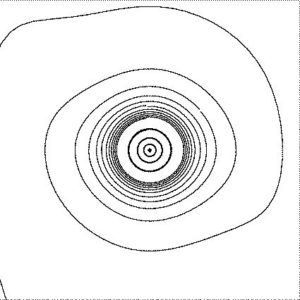# MATHEMATICS D (PDEs)

GENERAL DESCRIPTION OF THE COURSE

The course consists of two main parts: (systems of) ODEs (including some special families of ODEs and special functions, in particular Bessel functions and Dirac Delta and Laplace transform) and (linear) PDEs, including Fourier series (and Fourier transform) applications in mechanics. The calculations for the (individual) homework (see figure on the left below) are done with Scientific Notebook and Mathematica which is on this level very useful in power computing.

In case that less than 5 Erasmus students are enrolled, the students are invited to attend the course in Slovene language (where the lecturer emphasizes the important things in English language) and have weekly meetings with the lecturer and assistant. All materials for Erasmus students: Exam, (Individual) Homework exercises are in English language. Erasmus students have the same obligations as regular students. The students are invited to attend the tutorials to be prepared for the written exam.COURSE CONTENTS

The course will cover the following topics:

• Systems of ODEs: Theory of stability,
• Laplace transform
• Convolution and solutions of equations with non-smooth outer function
• Dirac Delta
• PDEs: Classification of PDEs of order two (elliptic, hyperbolic and parabolic equations),
• Fourier series, integrals and transforms
• Bessel functions,
• Wave and heat equation
• Dirichlet problem, Laplace equation.

STUDY MATERIALS:

• Kreyszig, Advanced Engineering Mathematics, John Wiley and Sons, 2006
• (https://www-elec.inaoep.mx/~jmram/Kreyzig-ECS-DIF1.pdf  mostly chapters 3-6 and part C)
• Polking, A. Boggess, D. Arnold, Differential equations with boundary value problems. Upper Saddle River, New Jersey, Pearson/Prentice Hall, 2006.
• B. Arfken, H. J. Weber, Mathematical methods for physicists. Harcourt/Academic Press, Burlington 2001.
• DuChateau, D. W. Zachmann, Schaum’s outline of theory and problems of partial differential equations. McGraw-Hill, New York, 2011.